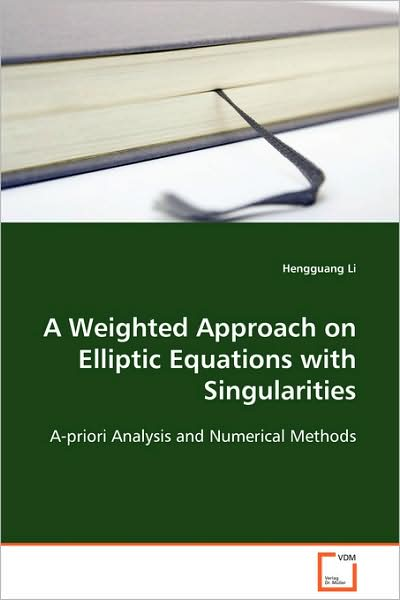## Hengguang Li

Pris
SEK 859
Förväntad leverans 23 jun - 3 jul

# A Weighted Approach on Elliptic Equations with Singularities: A-priori Analysis and Numerical Methods

Elliptic equations in a two- or three-dimensional bounded domain may have singular solutions from the non-smoothness of the domain, changes of boundary conditions, and discontinuities, singularities of the coefficients. These singularities give rise to various difficulties in the theoretical analysis and in the development of numerical algorithms for these equations. On the other hand, most of the problems arising from physics, engineering, and other applications have singularities of this form. In addition, the study on these elliptic equations leads to good understandings of other types of PDEs and systems of PDEs. This research, therefore, is not only of theoretical interest, but also of practical importance. This book includes a-priori estimates (well-posedness, regularity, and Fredholm property) for these singular solutions of general elliptic equations in weighted Sobolev spaces, as well as effective finite element schemes and corresponding multigrid estimates. Graduate students and researchers will find it a very useful introductory reference.

 Media Böcker     Pocketbok   (Bok med mjukt omslag och limmad rygg) Releasedatum 1 december 2008 ISBN13 9783639106091 Utgivare VDM Verlag Dr. Müller Antal sidor 160 Mått 222 g Språk Engelska